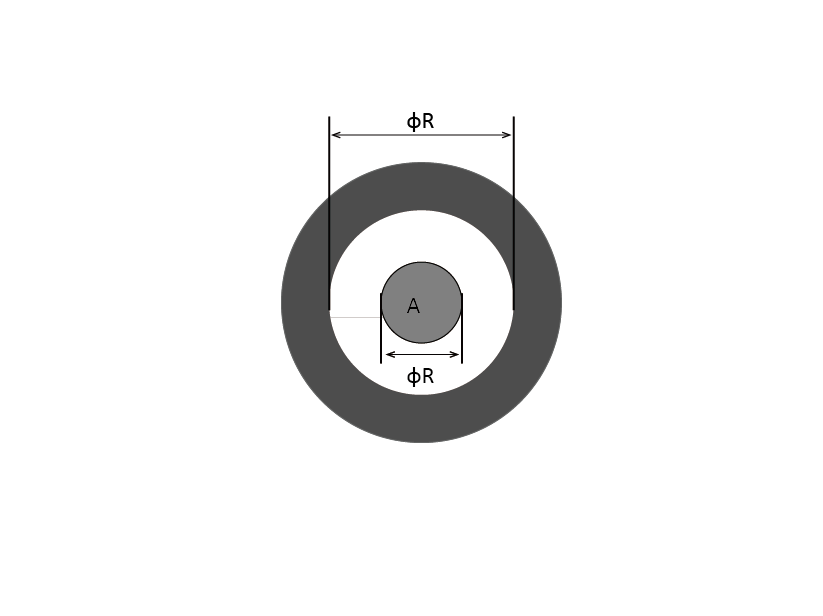Technology
Current Position: Home > Technology
置标出错:关键字 'and' 附近有语法错误。

# RF Performance

The characteristic impedance is complex and contains both resistance and reactance and is independent of length and frequency. The
characteristic impedance at high frequencies is defined by the voltage value of a single wave divided by the current value of a single
wave.
Since theinductance and capacitance are essentially independent of frequency in the “high” frequency range, in terms of inductance
and capacitance, characteristic impedance is the square root of the inductance per unit length (L) divided by the capacitance per unit length (C).Z= √L/C

L is determined by the ratio R/r
C is determined by the ratio R/r and ε
Where: r = Inside diameter of the shielding conductor
R = Outside diameter of the signal conductor
ε = Dielectric constant

As the spacing between R and r increases, in general, the inductance increases while the capacitance decreases. Therefore, the
characteristic impedance will decrease as the distance between the signal conductor and shielding tube decrease and increase when
this distance increases.

Company News
Company News Industry News New Product Infomation
Products
Pogo Pin ICT/FCT Probe
CSRF